a) What could be the maximum rank of A?
The maximum rank could not exceed the number of unknowns. So, the maximum rank is 3.
b) If the rank of A is equal to the number of unknowns, find the solution of the system. Write your answer in vector form.
The only solution of the system will be the trivial solution. Thus,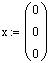c) If the rank of A is 1, how many free variables will be involved in the solution? Justify your answer.
number of free variables = 3 - 1 = 2
7. Let ei be the 2 x 1 i-th basic unit vector and let A be a 2 x 2 matrix such that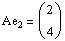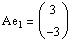Use Cramer's rule to solve the linear system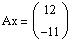Solution:
Using the property that Aei = the i-th column of A, we have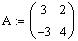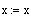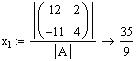(reinitialize the vector x)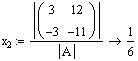Thus the solution is
Solution:
The only other method we know to compute the inverse of a matrix is to compute the reduced echelon form of the augmented matrix of of [ B | I ]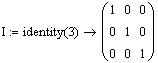(define I as the 3 x 3 identity matrix)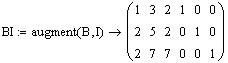(form the augmented matrix and name it BI)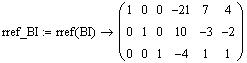(Compute the reduced row ech. form of BI)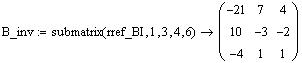(Extract the inverse from BI)
Check: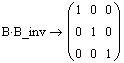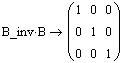c) Give your observation about the relationship between the rows of A and B and the columns of A-1 and B-1
(Display the matrices in question)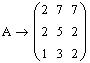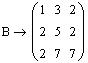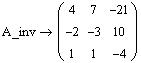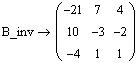We observe that B can be obtained form A by interchanging row 1 and row 3 of A,
and B-1 can be obtained form A-1 by interchanging column 1 and column 3 of A-1.
6. Consider the homogenous linear system Ax = 0. Let A be a 4 x 3 matrix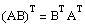==>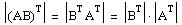(property of determinant of the product)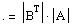==>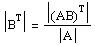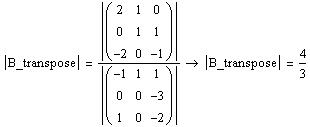b) find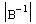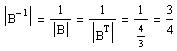11. Justify the following statements (give the reason why they are true)
a) Cramer's rule can't be used for solving non-square linear systems
Cramer's rule requires the use of determinants and determinants are not defined for non square matrices.
b) Homogenous linear systems have at least one solution
All homogeneous systems have the trivial solution
c) The inverse matrix method can't be used to solve the system Ax = b if | A | = 0.
If | A | = 0, then A is not invertible and the inverse matrix method requires the use of the inverse of A.
12. Answer the following questions by (T)rue or (F)alse
1. (F) If x and y are solutions to the nonhomogeneous system then their sum is a solution to the homogenous equation Ax = 0.

2. (T) If x and y are two solutions of the homogenous system Ax = 0 then their sum z = x + y is also a solution to the system.

3. (F) If A and B are square matrices and AB = AC, then B = C.

4. (T) If A and B are invertible matrices, then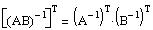5. (T) If A is invertible then the linear system Ax = 0 has only the trivial solution.

6. (F) A nonzero diagonal matrix is always invertible.

7. (T) If A is row equivalent to an identity matrix, then the linear system Ax = b has a unique solution.

8. (T) A nonhomogenous linear system with less equations than unknowns always has either no solution or infinite number of solutions.

9. (F) If the intersections of three lines in a plane form a triangle, then the linear system representing the three lines has three solutions.

10. (F) If the reduced row echelon form of the coefficient matrix of a nonhomogeneous linear system has a leading entry in the last column, then the system has no solution.

11. (F) If A is an n x n matrix then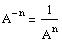.

12. (F) If a square matrix A is row equivalent to identity matrix I, then | A | = 1.

13. (F) If A and B are m x n matrices then, | A + B | = | A | + | B |.

14. (T) If A and B are m x n matrices then,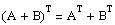.

15. (F) A square matrix A is orthogonal if A-1 = A.

16. (F) A linear system with less unknowns than equations is always consistent.

17. (F) If A is a square matrix with no zero rows or columns, then A is nonsingular.

18. (T) If A is invertible and AB = 0 then B = 0.

19. (F) If k is a nonzero constant, then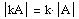.

20. (T) Students should work to earn their grades and not beg for them.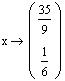8. Show that if x and y are two solutions of the nonhomogenous system Ax = b, then their difference z = x - y is a solution to the homogenous system Ax = 0.
Solution:
x is a solution ==> Ax = b

y is a solution ==> Ay = b

The sum x + y will be a solution to the homogenous system Ax = 0 if we can show that A(x + y) = 0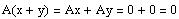Thus, x + y is a solution to the homogeneous system.
9. If a, b, and c are nonzero real numbers, find the inverse of the matrix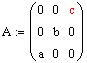Solution: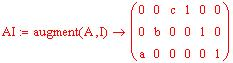(Augment A with the 3 x 3 Ident. matrix)
R1/c
R2/b == >
R3/a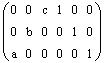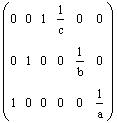R1 <--> R3 ==>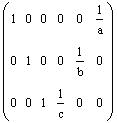Thus the inverse of A is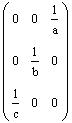10. if A and B are 3 x 3 matrices such that (AB)T =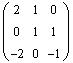and A =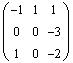a) find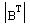(property of transpose of the product)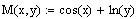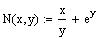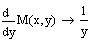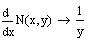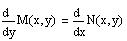==>
The equation is exact.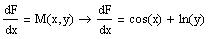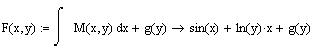(1)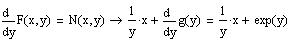Therefore,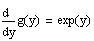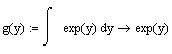Substitute in (1)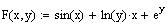Therefore, the solution is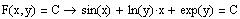3.
If possible, give examples of a reduced row echelon form of the augmented matrix of a linear system with the given conditions. If not possible, write "Impossible" as an answer.
Note: Use ' * ' to indicate that an element can be any real number
a) a nonhomogeneous system with 4 equations in 3 unknowns that has no solution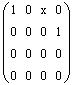Math260-032 (A. Farhat) Solution of Major Exam 1
2. Solve the initial value problem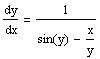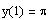Solution: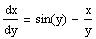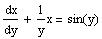(linear in x)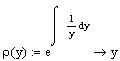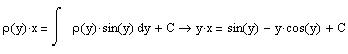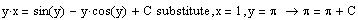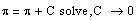Therefore, the solution is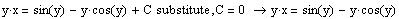Remark:
The problem can also be solved as an exact equation if rewritten in the form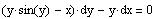2. Solve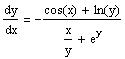Solution: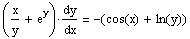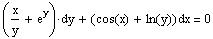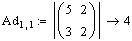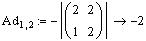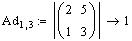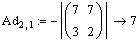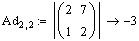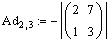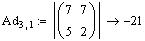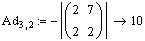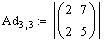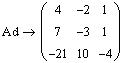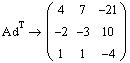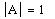(Compute the determinant of A)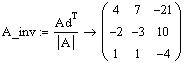(Compute the inverse of A and name it A_inv)
Check: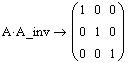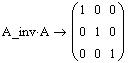b) Use another method to compute the inverse of the matrix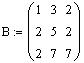or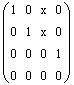Remark:
The matrix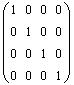i s not a valid answer because on one hand it says that we can solve for the tree unknowns (they are all zeros), and on the other hand it says that the system has no solution (due to the leading entry in the last column)

Another way to justify that it is a wrong answer is to compare the number of unknowns and the rank of the matrix. Here we have

Number of unknowns = 3, Rank = 4 ( not possible since the rank could never exceed the number of unknowns)

Alternatively,

# of free variable = # of unknowns - rank = 3 - 4 = -1 (not possible)
b) a nonhomogeneous system with 4 equations in 3 unknowns that has a unique solution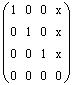c) a nonhomogeneous system with 4 equations in 3 unknowns that has infinite number of solutions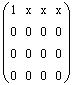or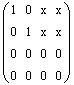or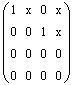d) a homogeneous system with 3 equations in 4 unknowns that has only the trivial solution
Not possible because the reduced row echelon form of the augmented matrix of a homogeneous system with 3 equations has a maximum rank equal to 3. And since

# of free variables = # of unknowns - rank

there will be at least one free variable.
5 a) Use the adjoint method to compute the inverse of the matrix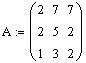Solution: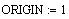(Set the starting subscript for Mathcad to 1)
Compute the cofactors as elements of a matrix named Ad